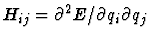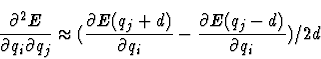Next: Implementation Up: The Free Format Section Previous: Control of constraints

## Calculation of the Hesse-Matrix

There are two keywords (HESSE and SELHESSE) which control the calculation of the cartesian force constant Hesse-Matrix. Such a Hesse-Matrix can be used later to calculate a IR vibrational spectrum. The approximation of the Hesse-Matrixis done by finite differences of the first derivatives of the total energy E. This is either done via a one point approximationor a two point approximation, whereas d denotes the step-length of each finite differene step in x, y and z direction. With the keyword HESSE one can select between one of the two modes and set the step length given in Å. The keyword SELHESSE is manditatory and is used to specify a set of atoms for which the Hesse-Matrix should be calculated. Example:
HESSE 2 0.01
SELHESSE A*
In that example all atoms are selected for the calculation of the Hesse-Matrix. The calculation is done by the two point approximation and uses a step length of
0.01Å. In the output-directory of EGO the file hesse.out is created with the corresponding Hesse-Matrix. The dimension of the elements in the Hesse-Matrix is N/m. In the util-directory there is the utiliy program hess2hess which is able to convert such a Hesse-Matrix to other formats and dimensions.

Note: Features which will affect the proper calculation of the Hesse-Matrix, like, e.g., Minimization, Equilibration or SHAKE are automatically switched off. Furthermore, the number of steps necessary to perform the calculation of the Hesse-Matrix is set automatically.

The Hesse-Matrix is stored in the file hesse.out. The header of that contains the atoms with their masses and positions. So, if you like to investigate isotipoc effects you just have to change the masses here in the file. After the keyword Matrix the hesse-matrix follows. Note: If you choose many atoms for a hesse-calulation the lines in that file get very long and some ASCII editors (like the good old vi will not work properly and will destroy the file).

It is also possible to restart a hesse calculation. Just restart again normally. EGO will look in the hesse.out file and will see how far it is. If it is not completed it will restart at the right point. EGO recognizes the status of the hesse-calcualtion from two numbers in the first line after the number of atoms. If it is not completed there will be for example 3 1 2 The first number specifies the number of atoms for which the hesse matrix should be calulated. The second number specifies the atom which is currently is moved and the third number specifies in which direction it is moved. Once it is completed only the number of atoms will be left.

Example for a completed hesse-matrix for H2O:
3
1  OH2       15.99940      -0.60543    0.00000    0.77339
2  H1         1.00800       0.00000    0.00000    1.54678
3  H2         1.00800       0.00000    0.00000    0.00000
Matrix:
620.76339    0.00555    0.12451   -302.92413    0.00365 -255.71554   -302.90517    0.00985  255.72955
1.34366  -18.29916   -0.03854     -0.53269   -2.53697   -0.13334     -0.52906   -2.54235    0.14224
-0.26595   -3.61815  867.78234   -346.08646   -0.31560 -440.00671    346.51745   -0.77901 -440.35201
-306.01033    0.02860 -349.09602    287.57882    0.00321  300.09174     15.91631   -0.01153   44.18106
4.88112   25.75767   -1.80326      1.59560    3.98306    0.36573     -0.07680   -3.65888   -0.43499
-256.41766    0.18216 -426.99687    301.58252    0.77197  486.95572    -45.46118   -1.13272  -46.69626
-306.92754    0.00193  359.79018     15.84619    0.01383  -43.98345    288.33121   -0.01516 -300.36040
4.50220   23.25857    1.67851     -0.02868   -2.56054    0.52004      1.64183    3.16829   -0.43899
256.58000    1.09238 -426.33925     45.55342   -0.96294  -46.79383   -301.54461    0.63691  487.03730Next: Implementation Up: The Free Format Section Previous: Control of constraints
Helmut Heller
2000-04-19